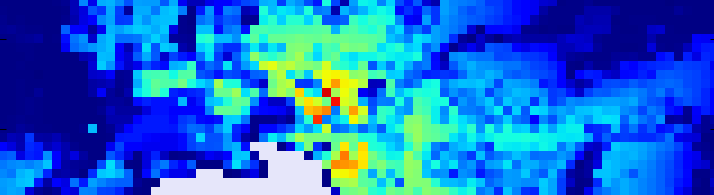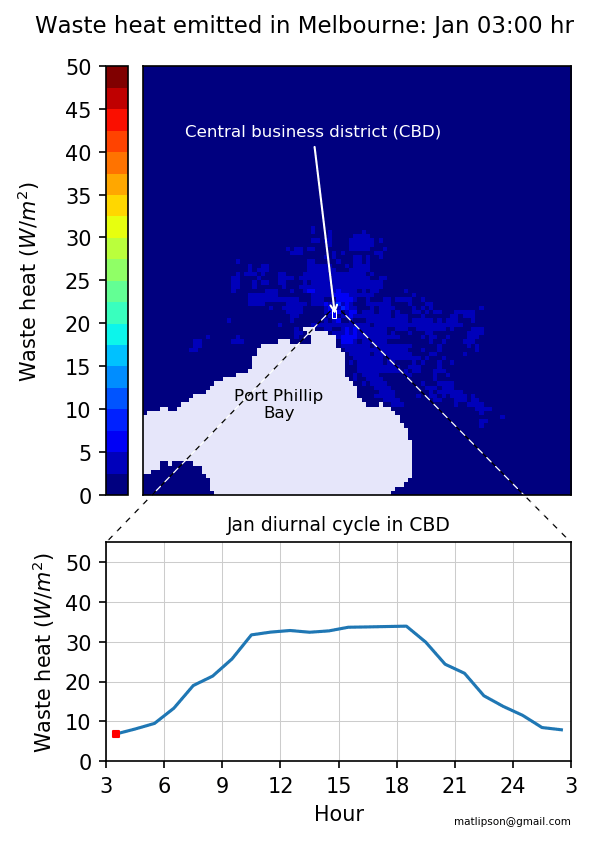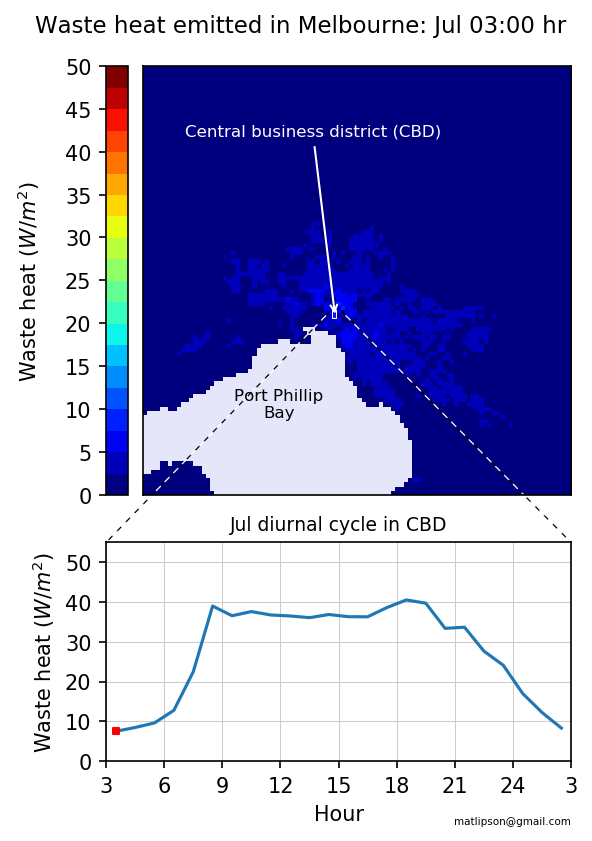# Waste heat in Melbourne

Cities can be much hotter than surrounding regions. This is in part because of waste heat from our energy use. This post shows how much waste heat is emitted during a typical day into Melbourne, Australia.

Most of the electricity and other forms of energy we use in cities ends up as waste heat. Whether it’s from car engines, air-conditioners, or heat leaking from buildings in the winter, our energy intensive activities can measurably increase temperatures in cities.

Here I’m plotting the intensity of waste heat based on a global dataset produced by a group from the Tokyo Institute of Technology. Dong et al. describe the dataset in a recent paper here. It’s a fantastic tool as the dataset has high spatial resolution (30 arc-seconds or about 1km) for every hour and for each month, over the entire globe.

The animation below cycles through a typical day in February (summer) for the Melbourne metropolitan area. Highlighted and plotted separately below is the central business district where the density of buildings results in the highest waste heat emission.You can see much more waste heat is being emitted during work hours, and that denser areas of the city emit more heat. In summer, heat emitted during the day is pretty constant, with a slight peak between 4-5pm when air-conditioning, peak-hour traffic and general electricity use combine.

Winter waste heat has a different diurnal profile:For the surrounding suburbs dominated by residential areas, peaks occur in the morning and early evening when people are waking up, commuting, or arriving home and heating their homes. For a cooler climate city like Melbourne, on average more heat is emitted during the winter than in the summer.

The peak values in the centre of Melbourne are about 50 $W/m^2.$ This is still small compared to other world cities reaching up to 500 $W/m^2$. The maximum summer midday sun in Australia at about 1000 $W/m^2$, so and you can appreciate that much extra energy our activities are pumping into the urban environment.

## Plotting the data

I use python for data analysis. I had to learn some new techniques to plot the data and create the animations in this post.

To create the gif animation, I found the package imageio very easy to use.

I had a bit of difficulty arranging the two plots and the colorbar together, so ended up using a helper function make_axes_locatable from mpl_toolkits.axes_grid1. This simplified the process of associating the size of the colorbar with the top axes only.

I also had difficulty drawing the zoom lines from one axes to the other until I came across the handy tool ConnectionPatch in matplotlib.patches.

As for the data, because of its high spatial and temporal resolution, the dataset is large, about 500 GB compressed. Instructions for download are here. Thanks to Alvin Varquez, one of the authors of the dataset, for asistance in seperating out the area around Melbourne.

The full plot code is available here.

import numpy as np
import matplotlib.pyplot as plt
from mpl_toolkits.axes_grid1 import make_axes_locatable
import matplotlib.patches as patches
import matplotlib.colors as colors
import imageio
import sys

melbm = {}
preston=np.zeros((12,24))
months = ['jan','feb','mar','apr','may','jun','jul','aug','sep','oct','nov','dec']
hours = ['03','04','05','06','07','08','09','10','11','12','13','14',
'15','16','17','18','19','20','21','22','23','00','01','02']

# set colormap colors
cmap=plt.get_cmap('jet')
bounds=np.linspace(0, 50, 41)
norm = colors.BoundaryNorm(boundaries=bounds, ncolors=cmap.N)

plt.close('all')
for i, month in enumerate(months):
animation = []
# populate arrays
for j, hour in enumerate(hours):
filename = '%s_ahe_%s' %(month,hour)
melb = melb[10:90,10:90]

preston[i,j] = melb[39:41,39:41].mean()
melbm['%s_%s' %(month,hour)] = np.ma.masked_where(melb < 0, melb)
# plot figure for month
for j, hour in enumerate(hours):
plt.close('all')
fig, axes = plt.subplots(ncols=1,nrows=2,
gridspec_kw = {'height_ratios':[2, 1], 'width_ratios':[1,1]})
# plot information
im = axes.imshow(melbm['%s_%s' %(month,hour)], norm=norm,cmap=cmap,
vmin=0, vmax=50, interpolation='nearest')
axes.plot([0.5+k for k in range(24)],preston[i,:])
axes.scatter(j+0.5,preston[i,j],marker='s',color='r',s=10)
# annotations
axes.set_title('Waste heat emitted in Melbourne: %s %s:00' %(month,hour),
fontsize=11,y=1.05,x=0.42)
axes.set_title('%s diurnal cycle in Preston' %month,fontsize=9,y=1.00,x=0.5)
axes.annotate('Preston',xy=(40,40), xytext=(40,10), color='red', fontsize=8,
ha='center',va='bottom', arrowprops={'arrowstyle':'->','color':'red'})
axes.text(15, 74,'Port Phillip Bay', fontsize=8,color='black')
axes.set_yticklabels([])
axes.set_xticklabels([])
# colour bar
divider = make_axes_locatable(axes)
fig.colorbar(im,cax=cax,norm=norm,boundaries=bounds,ticks=[0,10,20,30,40,50])
cax.yaxis.set_ticks_position('left')
cax.set_ylabel(r'Waste heat $(W/m^2)$',labelpad=-52)
# ticks and labels
axes.set_ylabel(r'Waste heat $(W/m^2)$')
axes.set_xlim(0,24)
axes.set_xticks([0,3,6,9,12,15,18,21,24])
axes.set_xticklabels([3,6,9,12,15,18,21,24,3])
axes.set_xlabel('Hour')
axes.set_ylim(0,25)
axes.set_yticks([0,5,10,15,20,25])
axes.grid(color='0.8')
for spine in axes.spines.values():
spine.set_edgecolor('red')
# zoom line
connect1 = patches.ConnectionPatch(xyA=(39,39), xyB=(0,25),coordsA='data',
coordsB='data', axesA=axes, axesB=axes,
color='red',linestyle=':',linewidth=0.6,shrinkA=2,shrinkB=2)
connect2 = patches.ConnectionPatch(xyA=(41,39), xyB=(24,25), coordsA='data',
coordsB='data', axesA=axes, axesB=axes,
color='red',linestyle=':',linewidth=0.6,shrinkA=2,shrinkB=2)
imageio.mimsave('plot/MelbourneQF_%s.gif' %(month), animation)